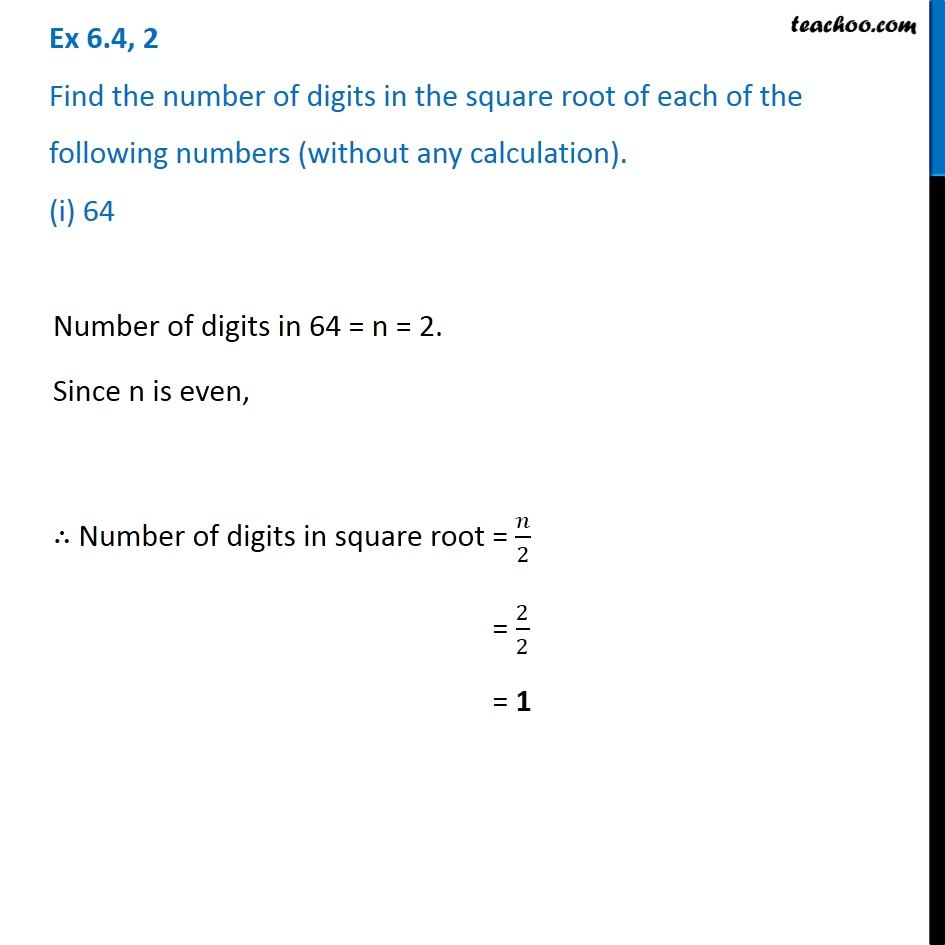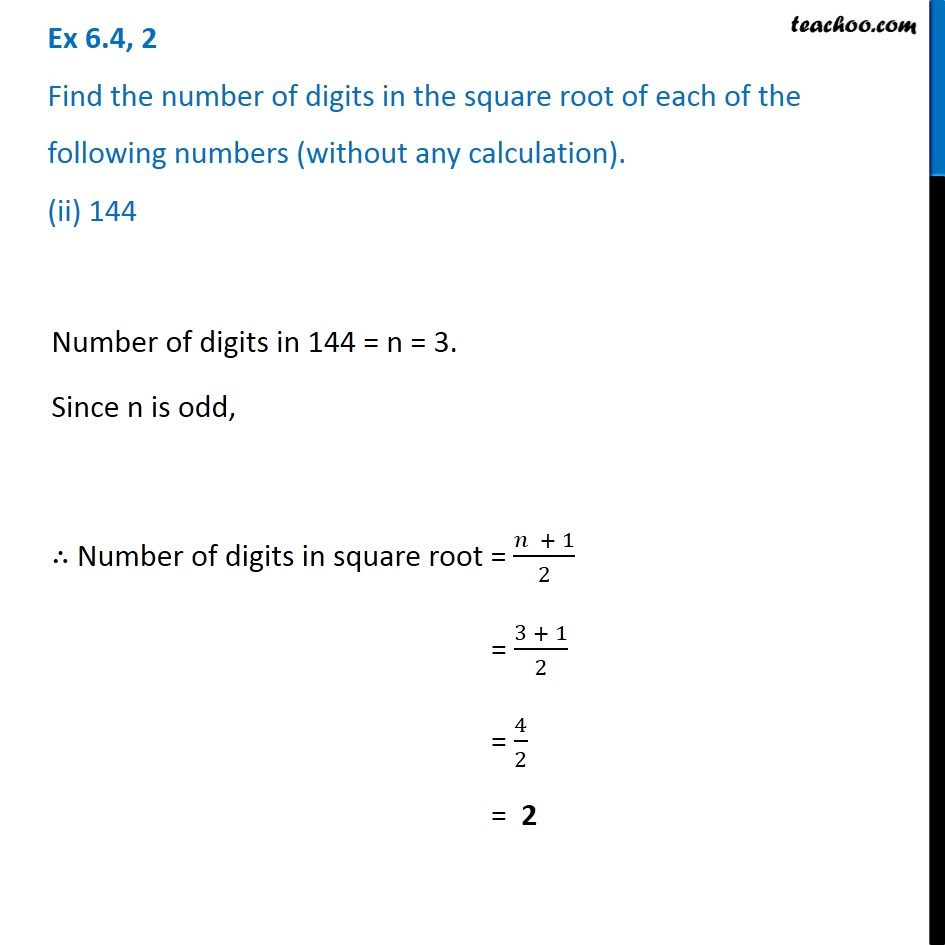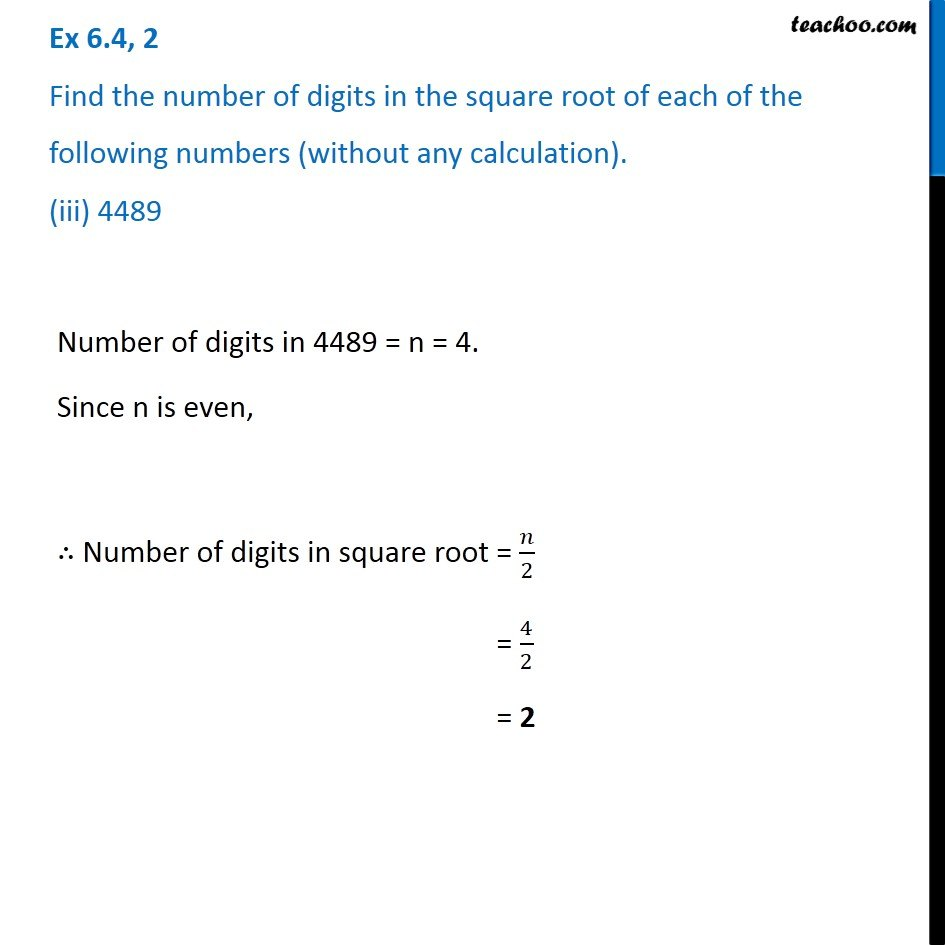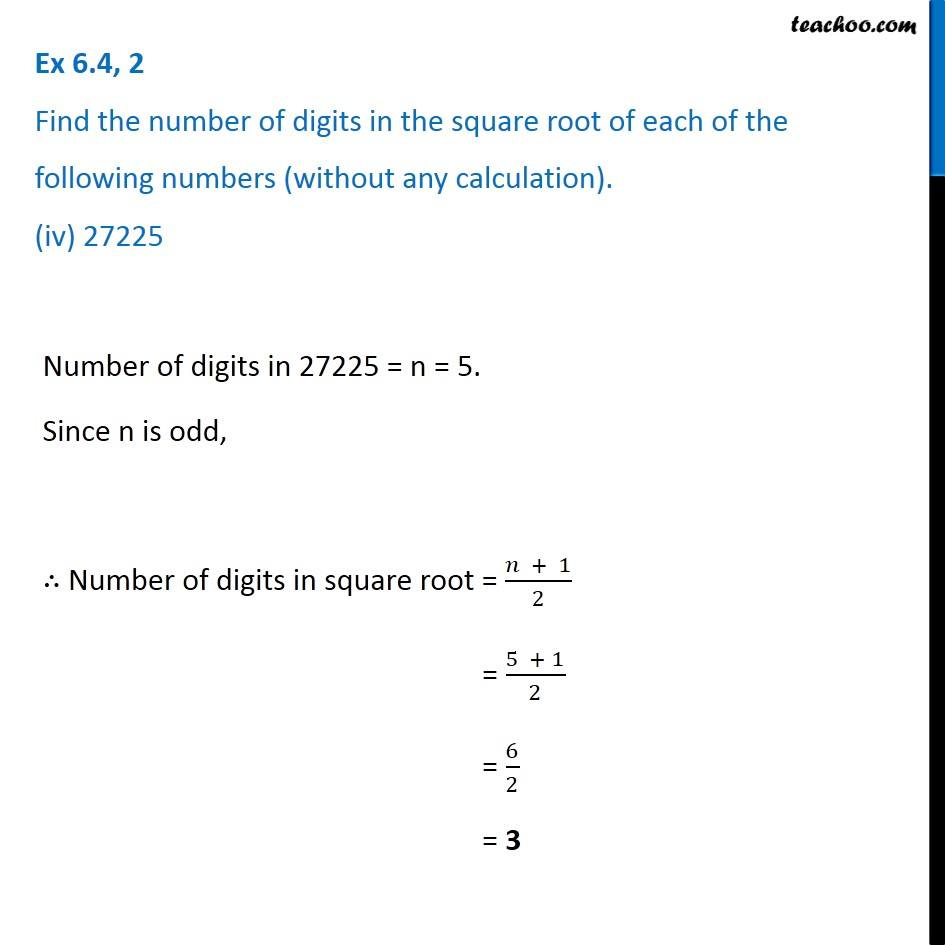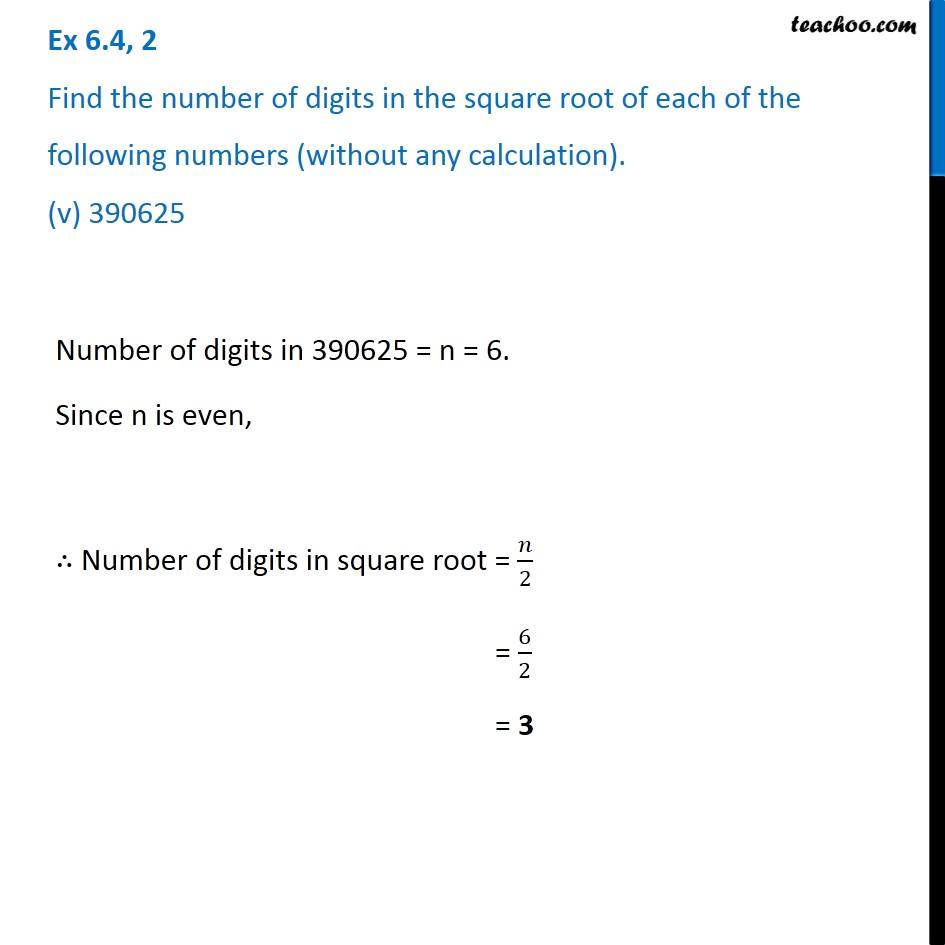Subscribe to our Youtube Channel - https://you.tube/teachoo

1. Chapter 6 Class 8 Squares and Square Roots
2. Concept wise
3. Finding number of digits in square root (without calculation)

Transcript

Ex 6.4, 2 Find the number of digits in the square root of each of the following numbers (without any calculation). (i) 64 Number of digits in 64 = n = 2. Since n is even, ∴ Number of digits in square root = 𝑛/2 = 2/2 = 1 Ex 6.4, 2 Find the number of digits in the square root of each of the following numbers (without any calculation). (ii) 144 Number of digits in 144 = n = 3. Since n is odd, ∴ Number of digits in square root = (𝑛 + 1)/2 = (3 + 1)/2 = 4/2 = 2 Ex 6.4, 2 Find the number of digits in the square root of each of the following numbers (without any calculation). (iii) 4489 Number of digits in 4489 = n = 4. Since n is even, ∴ Number of digits in square root = 𝑛/2 = 4/2 = 2 Ex 6.4, 2 Find the number of digits in the square root of each of the following numbers (without any calculation). (iv) 27225 Number of digits in 27225 = n = 5. Since n is odd, ∴ Number of digits in square root = (𝑛 + 1)/2 = (5 + 1)/2 = 6/2 = 3 Ex 6.4, 2 Find the number of digits in the square root of each of the following numbers (without any calculation). (v) 390625 Number of digits in 390625 = n = 6. Since n is even, ∴ Number of digits in square root = 𝑛/2 = 6/2 = 3

Finding number of digits in square root (without calculation)

About the AuthorDavneet Singh
Davneet Singh is a graduate from Indian Institute of Technology, Kanpur. He has been teaching from the past 9 years. He provides courses for Maths and Science at Teachoo.